## LSZ 约化公式

$\int d^4x e^{ip\cdot x} \langle \Omega|T\phi(x)\phi(0)|\Omega\rangle \underset{p^2\rightarrow m^2}{\sim} \frac{iZ}{p^2-m^2+i\epsilon}\tag{1}$

$\int d^4x e^{ip\cdot x} \langle \Omega|T\phi(x)\phi(z_1)\phi(z_2)\cdots|\Omega\rangle\tag{2}$

1. $x^0

2. $T_{-}

3. $x^0>T_{+}$

$\bm{1} = \sum_{\lambda} \int \frac{d^3q}{(2\pi)^3}\frac{1}{2E_{\bm{q}}(\lambda)}|\lambda_{\bm{q}}\rangle\langle\lambda_{\bm{q}}|$

\begin{aligned} &\int_{T_{+}}^{\infty}dx^0 \int d^3x e^{ip^0x^0}e^{-i\bm{p}\cdot\bm{x}}\sum_{\lambda} \int \frac{d^3q}{(2\pi)^3}\frac{1}{2E_{\bm{q}}(\lambda)}\langle\Omega|\phi(x)|\lambda_{\bm{q}}\rangle\langle\lambda_{\bm{q}}|T\{\phi(z_1)\phi(z_2)\cdots\}|\Omega\rangle\\ =& \sum_{\lambda}\int_{T_{+}}^{\infty}dx^0 \int \frac{d^3q}{(2\pi)^3}\frac{1}{2E_{\bm{q}}(\lambda)}e^{ip^0x^0}e^{-iq^0x^0}e^{-\epsilon x^0} \langle \Omega|\phi(0)|\lambda_0\rangle(2\pi)^3\delta^{(3)}(\bm{p}-\bm{q})\langle\lambda_{\bm{q}}|T\{\phi(z_1)\phi(z_2)\cdots\}|\Omega\rangle\\ =& \sum_{\lambda} \frac{1}{2E_{\bm{p}}(\lambda)}\frac{ie^{i(p^0-E_{\bm{p}}+i\epsilon)T_+}}{p^0-E_{\bm{p}}(\lambda)+i\epsilon}\langle\Omega|\phi(0)|\lambda_0\rangle\langle\lambda_{\bm{q}}|T\{\phi(z_1)\phi(z_2)\cdots\}|\Omega\rangle\\ \end{aligned}

$\int d^4x e^{ip\cdot x} \langle \Omega|T\phi(x)\phi(z_1)\phi(z_2)\cdots|\Omega\rangle \underset{p^0\rightarrow + E_{\bm{p}}}{\sim} \frac{i}{p^2-m^2+i\epsilon}\sqrt{Z}\langle\bm{p}|T\{\phi(z_1)\cdots\}|\Omega\rangle\tag{3}$

$\int d^4x e^{ip\cdot x} \langle \Omega|T\phi(x)\phi(z_1)\phi(z_2)\cdots|\Omega\rangle \underset{p^0\rightarrow - E_{\bm{p}}}{\sim} \langle\Omega|T\{\phi(z_1)\cdots\}|-\bm{p}\rangle\sqrt{Z}\frac{i}{p^2-m^2+i\epsilon}\tag{4}$

$\int d^4x e^{ip^0x^0}e^{-i\bm{p}\cdot\bm{x}}\rightarrow \int \frac{d^3k}{(2\pi)^3} \int d^4x e^{ip^0x^0}e^{-i\bm{k}\cdot\bm{x}}\varphi(\bm{k})\tag{5}$

$\varphi(\bm{k})$ 退化为 $\delta$ 函数时，就回到了傅里叶变换的情形。如此 $(3)$ 式成为：

\begin{aligned} &\int \frac{d^3k}{(2\pi)^3} \int d^4x e^{ip^0x^0}e^{-i\bm{k}\cdot\bm{x}}\varphi(\bm{k}) \langle \Omega|T\phi(x)\phi(z_1)\phi(z_2)\cdots|\Omega\rangle\\ =& \sum_{\lambda}\int \frac{d^3k}{(2\pi)^3} \varphi(\bm{k}) \frac{1}{2E_{\bm{k}}(\lambda)} \frac{i}{ p^0 - E_{\bm{k}}(\lambda)+ i\epsilon}\langle \Omega|\phi(0)|\lambda_0\rangle\langle \lambda_{\bm{k}}|T\{\phi(z_1)\cdots\}|\Omega\rangle \\ \underset{p^0\rightarrow + E_{\bm{p}}}{\sim} & \int \frac{d^3k}{(2\pi)^3} \varphi(\bm{k})\frac{i}{\tilde{p}^2-m^2+i\epsilon}\sqrt{Z}\langle\bm{k}|T\{\phi(z_1)\cdots\}|\Omega\rangle\\ \end{aligned}

$(\prod_i\int\frac{d^3k_i}{(2\pi)^3}\int d^4x_i e^{i \tilde{p}_i\cdot x_i}\varphi_i(\bm{k}_i))\langle\Omega|T\{\phi(x_1)\phi(x_2)\cdots\}|\Omega\rangle \tag{6}$

1. $x_i^0

2. $T_{-}

3. $x_i^0>T_{+}$

$T\{\phi(x_1)\phi(x_2)\phi(x_3)\cdots\} = T\{\phi(x_1)\phi(x_2)\}T\{\phi(x_3)\cdots\}$

$(\bm{1})_{two\ particles} = \sum_{\lambda} \int \frac{d^3K}{(2\pi)^3}\frac{1}{2E_{\bm{K}}}|\lambda_{\bm{K}}\rangle\langle\lambda_{\bm{K}}|$

\begin{aligned} \sum_{\lambda} \int \frac{d^3K}{(2\pi)^3}\frac{1}{2E_{\bm{K}}} (&\prod_{i=1,2}\int\frac{d^3k_i}{(2\pi)^3}\int d^4x_i e^{i \tilde{p}_i\cdot x_i}\varphi_i(\bm{k}_i))\\ &\times \langle\Omega|T\{\phi(x_1)\phi(x_2)\}|\lambda_{\bm{K}}\rangle\langle\lambda_{\bm{K}}|T\{\phi(x_3)\cdots\}|\Omega\rangle\\ \end{aligned}\tag{7}

$\lambda_{\bm{K}}$ 含有的两个粒子是相互分离，彼此独立的两个态。那么我们可以把 $\lambda_{\bm{K}}$ 写为两个单粒子态的直积态的形式：

$|\lambda_{\bm{K}}\rangle = |\lambda_{\bm{q}_1}\rangle|\lambda_{\bm{q}_2}\rangle$

\begin{aligned} &\sum_{\lambda_1,\lambda_2} \int \frac{d^3q_1}{(2\pi)^3}\frac{1}{2E_{\bm{q_1}}}\int \frac{d^3q_2}{(2\pi)^3}\frac{1}{2E_{\bm{q_2}}} (\prod_{i=1,2}\int\frac{d^3k_i}{(2\pi)^3}\int d^4x_i e^{i \tilde{p}_i\cdot x_i}\varphi_i(\bm{k}_i))\\ &\times \langle\Omega|\phi(x_1)|\lambda_{\bm{q_1}}\rangle\langle\Omega|\phi(x_1)|\lambda_{\bm{q_2}}\rangle\langle\lambda_{\bm{q}_1}\lambda_{\bm{q}_2}|T\{\phi(x_3)\cdots\}|\Omega\rangle\\ \underset{\bm{k}_i^0\rightarrow E_{\bm{k}_i}}{\sim}& (\prod_{i=1,2} \int \frac{d^3k_i}{(2\pi)^3}\varphi_i(\bm{k}_i)\frac{i}{\tilde{p}_i^2-m^2+i\epsilon}\cdot \sqrt{Z})\langle \bm{k}_1\bm{k}_2|T\{\phi(x_3)\cdots\}|\Omega\rangle\\ \end{aligned}\tag{8}

$(\prod_{i=1,2} \frac{i}{p_i^2-m^2+i\epsilon}\cdot \sqrt{Z})\langle \bm{p}_1\bm{p}_2|T\{\phi(x_3)\cdots\}|\Omega\rangle$

$(\prod_{i=1,2} \frac{i}{p_i^2-m^2+i\epsilon}\cdot \sqrt{Z})(\prod_{i=3,\cdots} \frac{i}{p_i^2-m^2+i\epsilon}\cdot \sqrt{Z})_{out}\langle \bm{p}_1\bm{p}_2|-\bm{p}_3\cdots\rangle_{in}$

\begin{aligned} &\prod_{1}^{n}\int d^4x_i e^{ip_i\cdot x_i} \prod_{1}^{m}\int d^4y_i e^{-ik_i\cdot y_i}\langle\Omega|T\{\phi(x_1)\cdots \phi(x_n)\phi(y_1)\cdots \phi(y_m)\}|\Omega\rangle\\ \underset{\bm{p}_i^0\rightarrow E_{\bm{p}_i},\bm{k}_i^0\rightarrow E_{\bm{k}_i}}{\sim}& (\prod_{i=1}^{n} \frac{\sqrt{Z} i}{p_i^2-m^2+i\epsilon})(\prod_{j=1}^{m} \frac{\sqrt{Z} i}{k_j^2-m^2+i\epsilon})\langle \bm{p}_1\cdots \bm{p}_n|S|\bm{k}_1\cdots\bm{k}_m\rangle \tag{9} \end{aligned}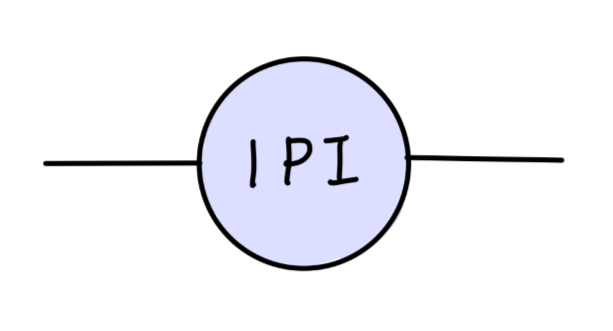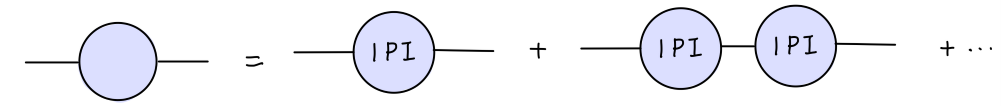$\frac{i}{p^2 - m_0^2 - M^2(p^2)} \underset{p^0\rightarrow E_{\bm{p}}}{\sim} \frac{iZ}{p^2-m^2}$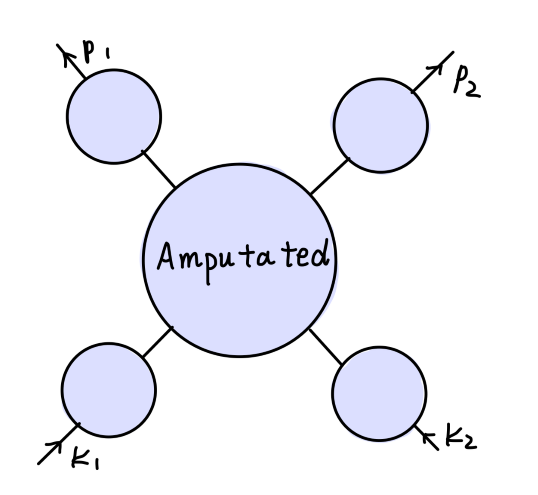$\frac{iZ}{p_1^2-m^2}\frac{iZ}{p_2^2-m^2}\frac{iZ}{k_1^2-m^2}\frac{iZ}{k_2^2-m^2}$

$\langle \bm{p}_1\bm{p}_2|S|\bm{k}_1\bm{k}_2\rangle = (\sqrt{Z})^{4}i\mathcal{M}_{amputated}$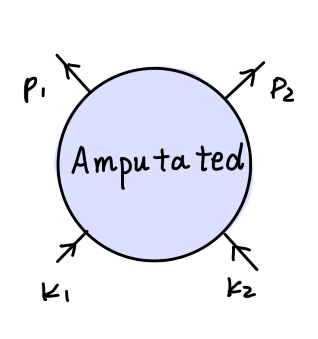## 光学定理

\begin{aligned} S &= 1+iT\\ -i(T-T^\dagger) &= T^\dagger T\\ \end{aligned}

$-i[\mathcal{M}(a\rightarrow b) - \mathcal{M}^*(b\rightarrow a)] = \sum_f \int d\Pi_f \mathcal{M}^*(b\rightarrow f)\mathcal{M}(a\rightarrow f)\tag{10}$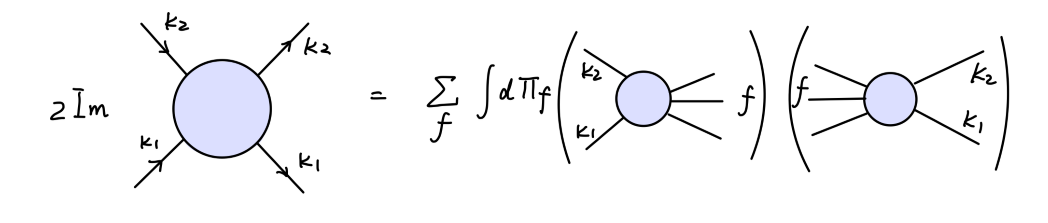$\mathcal{M}(s) = [\mathcal{M}(s^*)]^*\tag{11}$

\begin{aligned} &\mathrm{Re}\mathcal{M}(s+i\epsilon) = \mathrm{Re}\mathcal{M}(s-i\epsilon)\\ &\mathrm{Re}\mathcal{M}(s+i\epsilon) = - \mathrm{Im}\mathcal{M}(s-i\epsilon)\\ \end{aligned}\tag{12}

\begin{aligned} \mathrm{Disc}\mathcal{M}(s) &= \mathcal{M}(s+i\epsilon) - \mathcal{M}(s-i\epsilon)\\ &= 2i\mathrm{Im}\mathcal{M}(s+i\epsilon)\\ \end{aligned}\tag{13}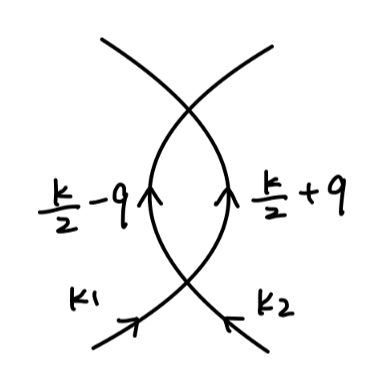\begin{aligned} i\delta \mathcal{M} = \frac{\lambda^2}{2}\int \frac{d^4q}{(2\pi)^4} \frac{1}{(k/2-q)^2 - m^2 + i\epsilon}\frac{1}{(k/2+q)^2 - m^2 + i\epsilon}\tag{14} \end{aligned}

\begin{aligned} q^0 = \frac{1}{2}k^0 \pm (E_{\bm{q}} -i\epsilon)\\ q^0 = -\frac{1}{2}k^0 \pm (E_{\bm{q}} -i\epsilon)\\ \end{aligned}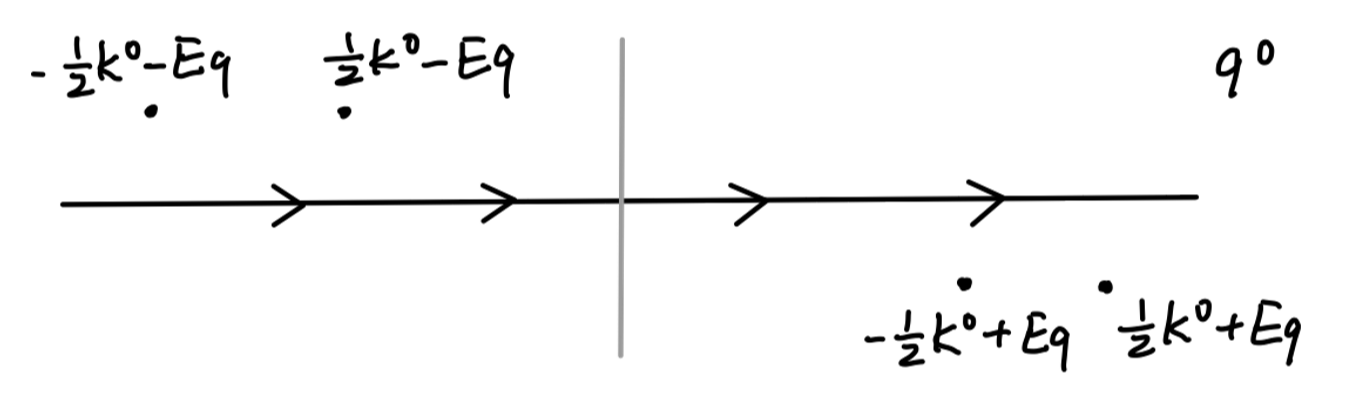$q^0 = \pm\frac{1}{2}k^0 + E_{\bm{q}} -i\epsilon$

\begin{aligned} i\delta \mathcal{M} &= -2\pi i\frac{\lambda^2}{2}\int \frac{d^3q}{(2\pi)^4}\frac{1}{2E_{\bm{q}}}\frac{1}{(k^0-E_{\bm{q}})^2 - E_{\bm{q}}^2}\\ &= -2\pi i\frac{\lambda^2}{2}\int \frac{d^3q}{(2\pi)^4}\frac{1}{2E_{\bm{q}}}\frac{1}{(k^0-E_{\bm{q}})^2 - E_{\bm{q}}^2}\\ &= -2\pi i\frac{\lambda^2}{2}\frac{4\pi}{(2\pi)^4}\int_{m}^{\infty} dE_{\bm{q}}E_{\bm{q}}|\bm{q}| \frac{1}{2E_{\bm{q}}}\frac{1}{k^0(k^0-2E_{\bm{q}})}\\ \end{aligned}\tag{15}

$\frac{1}{(k/2+q)^2-m^2+i\epsilon} \rightarrow -2\pi i\delta((k/2+q)^2-m^2)$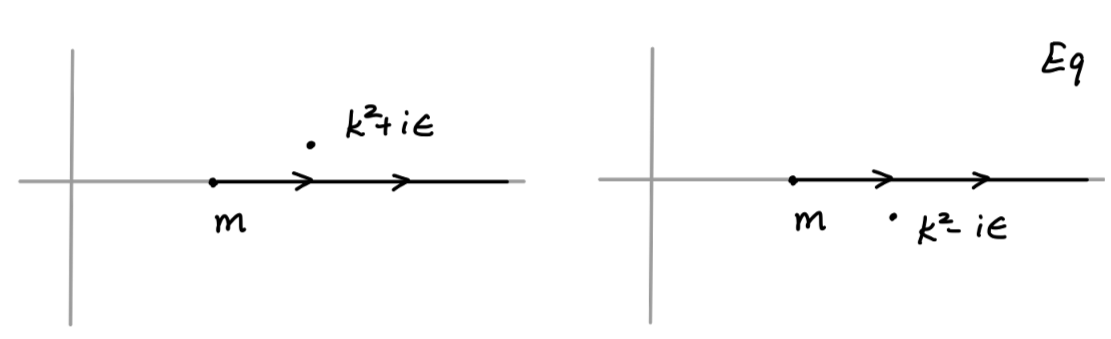$\frac{1}{k^0 - 2E_{\bm{q}} \pm i\epsilon} = P\frac{1}{k^0 - 2E_{\bm{q}}} \mp i\pi \delta(k^0-2E_{\bm{q}})$

$\frac{1}{(k/2-q)^2-m^2+i\epsilon} \rightarrow -2\pi i\delta((k/2-q)^2-m^2)$

$i\delta \mathcal{M} = \frac{\lambda^2}{2}\int \frac{d^4p_1}{(2\pi)^4}\int \frac{d^4p_2}{(2\pi)^4} \frac{1}{p_1^2 - m^2 + i\epsilon}\frac{1}{p_2^2 - m^2 + i\epsilon}\delta^{(4)}(p_1+p_2-k)$

$\frac{1}{p_i^2-m^2+i\epsilon} \rightarrow -2\pi i\delta(p_i^2-m^2)\tag{16}$

$i\delta \mathcal{M} = -\frac{\lambda^2}{2}\int \frac{d^3p_1}{(2\pi)^3}\frac{1}{2E_1} \int \frac{d^3p_2}{(2\pi)^3} \frac{1}{2E_2}\delta^{(4)}(p_1+p_2-k)$

$|\mathcal{M}(k)|^2 = \lambda^2$

$\mathrm{Disc}\mathcal{M}(k) = \frac{i}{2}\int \frac{d^3p_1}{(2\pi)^3}\frac{1}{2E_1} \int \frac{d^3p_2}{(2\pi)^3} \frac{1}{2E_2} |\mathcal{M}(k)|^2 \delta^{(4)}(p_1+p_2-k)$

Cutkosky 证明了如下计算不连续性的普遍方法：

1. 将费曼图进行所有可能的分割，使得被分割的传播子能够同时在壳。
2. 对于每一个被分割的传播子，做替代 $1/(p_i^2-m^2+i\epsilon) \rightarrow -2\pi i\delta(p_i^2-m^2)$
3. 将所有可能的分割方式的贡献求和。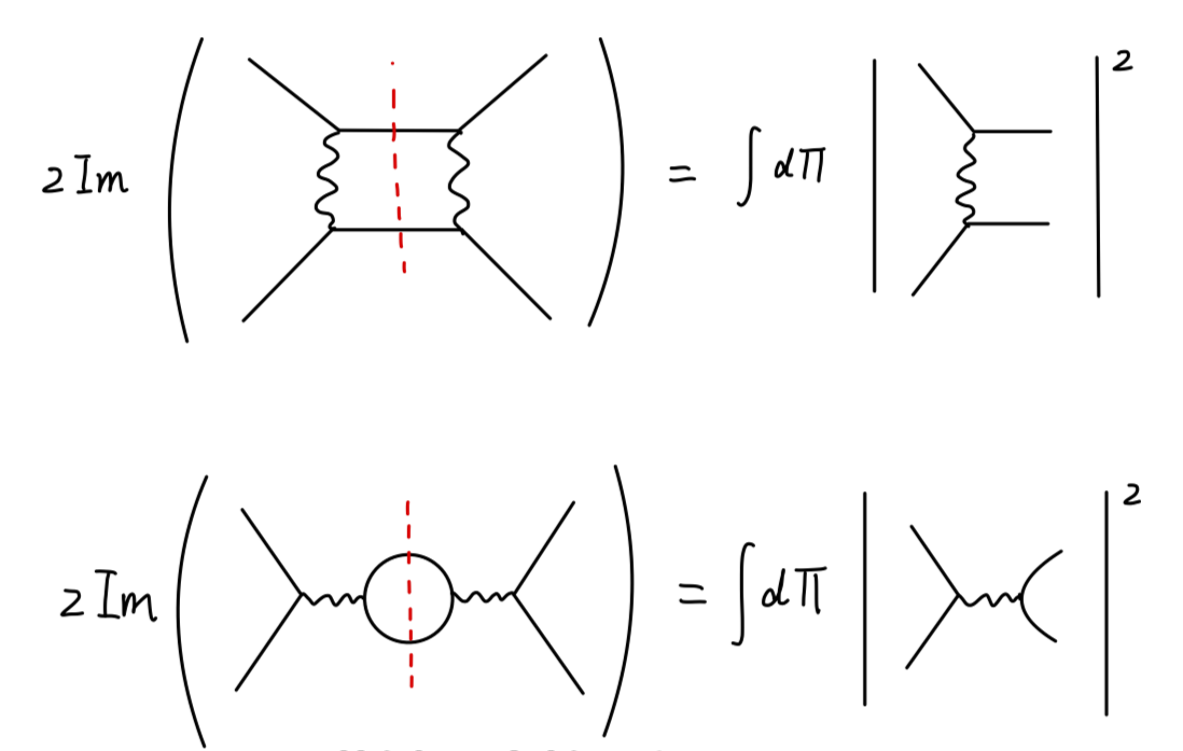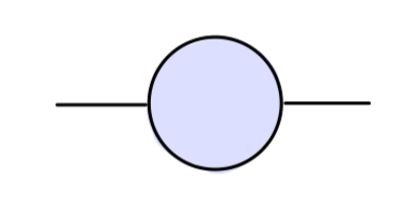$\frac{i}{p^2-m_0^2-M^2(p^2)}$

$\mathcal{M}(p\rightarrow p) = -ZM^2(p^2)\tag{17}$

$m^2 - m_0^2 -M^2(m^2) = 0 \tag{18}$

$m^2 - m_0^2 - \mathrm{Re} M^2(m^2) = 0 \tag{19}$

$\frac{iZ}{p^2-m^2 - iZ\mathrm{Im}M^2(p^2)} \tag{20}$

$\sigma\propto |\frac{1}{s-m^2-iZ\mathrm{Im}M^2(p^2)}|$

$\sigma \propto |\frac{1}{p^2-m^2+im\Gamma}|^2$

$\Gamma = -\frac{Z}{m}\mathrm{Im}M^2(m^2) \tag{21}$

$\Gamma = \frac{1}{2m}\sum_f\int d\Pi_f |\mathcal{M}(p\rightarrow f)|^2 \tag{22}$